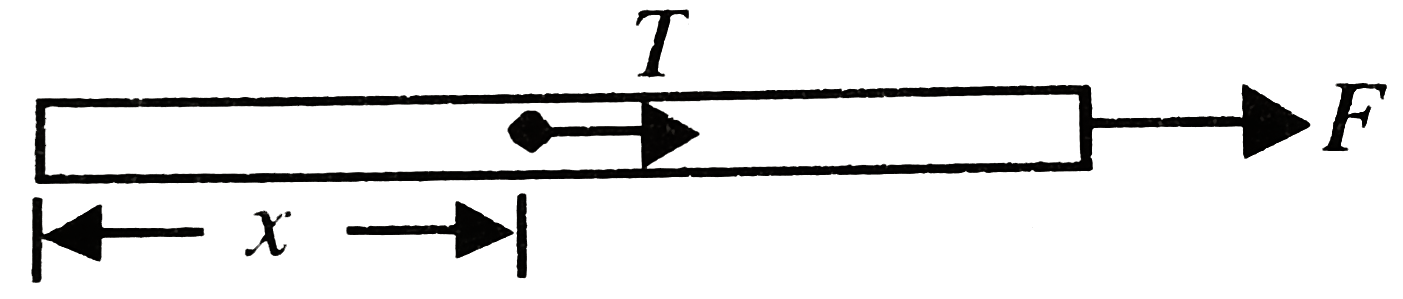# A smooth uniform, string of natural length l, cross-sectional area A and Young’s modulus Y is pulled along its length by a force F on a horizo

308 views
in Physics
closed
A smooth uniform, string of natural length l, cross-sectional area A and Young’s modulus Y is pulled along its length by a force F on a horizontal surface. Find the elastic potential energy stored in the string.

by (87.1k points)
selected

Correct Answer - U=(F^(2)l)/(6AY)
As discussed Mechanics the tension T in the string at a distance x from its free end is given
T=F/lx
Hence stress, p=T/A=F/(Al)xSubstituting p in the formulaU=1/(2Y)intp^(2)dV
we have U=1/(2Y)int_(0)^(l) (F^(2))/(A^(2)l^(2))x^(2)dV, where dV=Adx
This gives U=(F^(2)l)/(6AY)